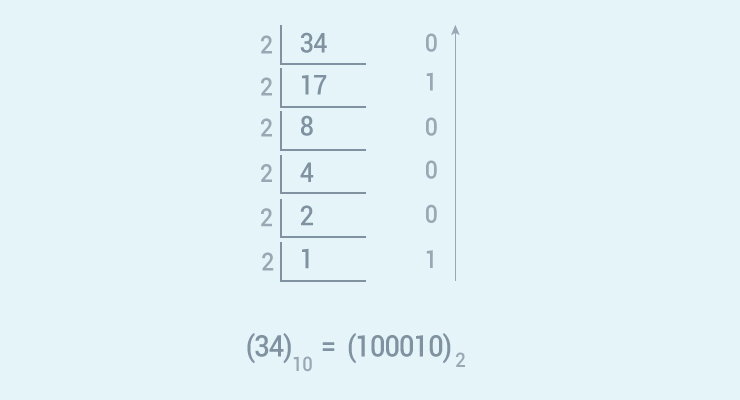Python Program to Convert Decimal to Binary Using Recursion

# Python Program to Convert Decimal to Binary Using Recursion

#### In this program, you will learn to convert decimal number to binary using recursive function.

To understand this example, you should have the knowledge of the following Python programming topics:

Decimal number is converted into binary by dividing the number successively by 2 and printing the remainder in reverse order.## Source Code

``````# Function to print binary number using recursion
def convertToBinary(n):
if n > 1:
convertToBinary(n//2)
print(n % 2,end = '')

# decimal number
dec = 34

convertToBinary(dec)
print()
``````

Output

```110100
```

You can change the variable dec in the above program and run it to test out for other values.

This program works only for whole numbers. It doesn't work for real numbers having fractional values such as: 25.5, 45.64 and so on. We encourage you to create Python program that converts decimal numbers to binary for all real numbers on your own.